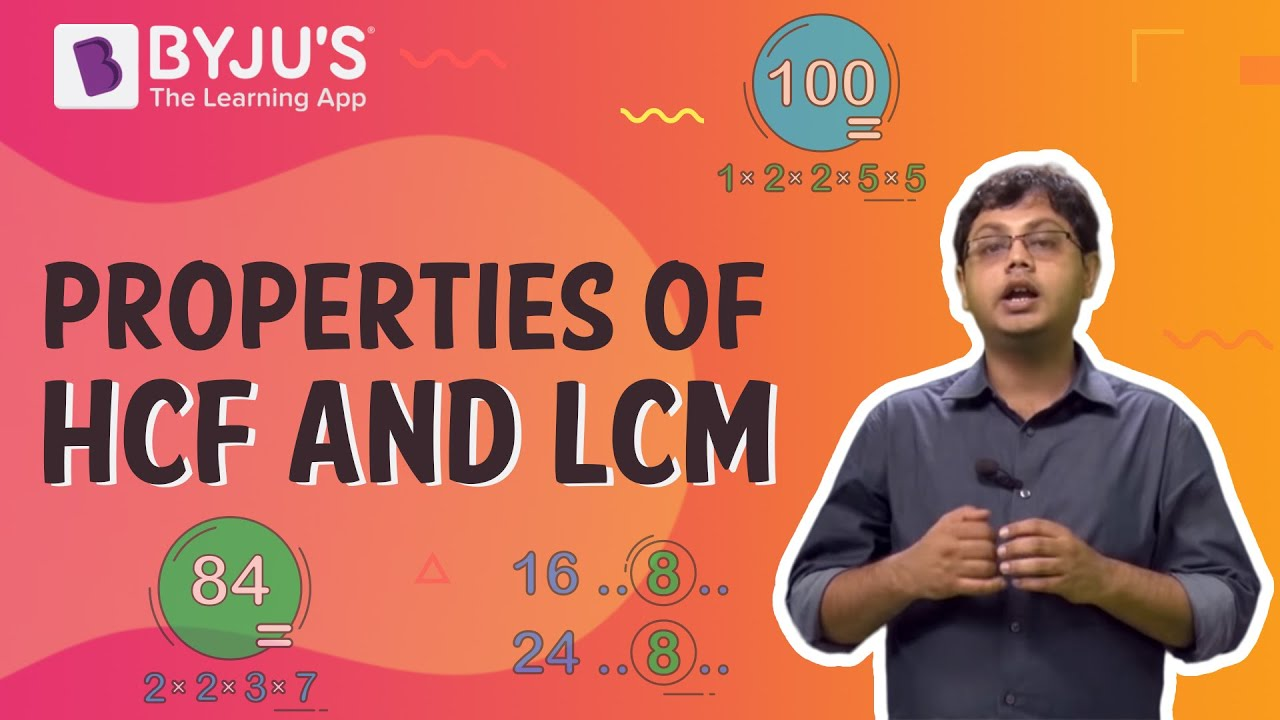# What is the HCFof 30 and 42?

The Highest Common Factor (HCF) of two or more numbers is the greatest of all their common factors. Therefore, it is also called the greatest common factor (GCF)

### What is HCF?

The full form of HCF in Maths is Highest Common Factor.

HCF is defined as the greatest common divisor or the gcd of two or more positive integers happens to be the largest positive integer that divides the numbers without leaving a remainder.

### Solution

List out all the factors of the given numbers.

The factors of number 30 are 1, 2, 3, 5, 6, 10, 15 and 30

The factors of a number 42 are 1, 2, 3, 6, 7, 14, 21 and 42

The highest common factor of 30 and 42 is 6.

Check out the video given below to know more about HCF and LCMArticles to explore

1. Find LCM and HCF of 510 and 92? Verify LCM and HCF is equal to the product of two numbers.(6)(1)